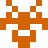### Real Vim ninjas count every keystroke - do you?

###### Pick a challenge, fire up Vim, and show us what you got.

```Your VimGolf key: please sign in

\$ gem install vimgolf
\$ vimgolf setup
\$ vimgolf put 50c195c1b85576000200005b
```

### fib.c cleanup

cleanup the file

##### Start file
```/* Fibonacci Series c language */
#include<stdio.h>

main()
{
int n, first = 0, second = 1, next, c;

printf("Enter the number of terms\n");
scanf("%d",&n);

printf("First %d terms of Fibonacci series are :-\n",n);

for ( c = 0 ; c < n ; c++ )
{
if ( c <= 1 )
next = c;
else
{
next = first + second;
first = second;
second = next;
}
printf("%d\n",next);
}

return 0;
}
```
##### End file
```/* Fibonacci Series c language */
#include<stdio.h>

main()
{
int n, first = 0, second = 1, next, c;

printf("Enter the number of terms\n");
scanf("%d",&n);

printf("First %d terms of Fibonacci series are :-\n",n);

for ( c = 0 ; c < n ; c++ )
{
if ( c <= 1 )
next = c;
else
{
next = first + second;
first = second;
second = next;
}
printf("%d\n",next);
}

return 0;
}
```

#### View Diff

```[1;33mdiff --git a/input.txt b/output.txt[m
[1;33mindex 2256c5a..aeb1794 100644[m
[1;33m--- a/input.txt[m
[1;33m+++ b/output.txt[m
[1;35m@@ -1,27 +1,27 @@[m
/* Fibonacci Series c language */[m
#include<stdio.h>[m

main()[m
{[m
int n, first = 0, second = 1, next, c;

printf("Enter the number of terms\n");
scanf("%d",&n);

printf("First %d terms of Fibonacci series are :-\n",n);

for ( c = 0 ; c < n ; c++ )
{
if ( c <= 1 )
next = c;
else
{
next = first + second;
first = second;
second = next;
}
printf("%d\n",next);
}

return 0;
}[m
```

### Solutions by @Xelrifos:

Unlock 1 remaining solutions by signing in and submitting your own entry

## 53 active golfers, 65 entries

##### Solutions by @Xelrifos:16
###### #34 - Xelrifos / @Xelrifos

02/23/2014 at 12:41PM